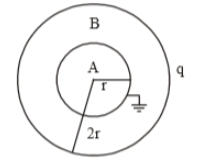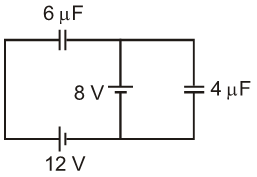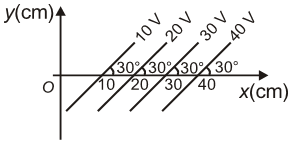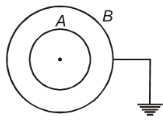The electric potential at a point at distance 'r' from a short dipole is proportional to

(1) ${r}^{2}$

(2) ${\mathrm{r}}^{-1}$

(3) ${r}^{-2}$

(4) ${r}^{1}$

Subtopic:  Electric Potential |
To view explanation, please enrol in the course below.
NEET 2021 - Achiever Batch - Aryan Raj Singh

Difficulty Level:

A hollow charged metal spherical shell has radius R. If the potential difference between its surface and a point at a distance 3R from the center is V, then the value of electric field intensity at a point at distance 4R from the center is

1.  $\frac{3\mathrm{V}}{19\mathrm{R}}$

2.  $\frac{\mathrm{V}}{6\mathrm{R}}$

3.  $\frac{3\mathrm{V}}{32\mathrm{R}}$

4.  $\frac{3\mathrm{V}}{16\mathrm{R}}$

Subtopic:  Electric Potential |
To view explanation, please enrol in the course below.
NEET 2021 - Achiever Batch - Aryan Raj Singh

Difficulty Level:

Capacitors  are connected in series across a source of emf 20KV. The potential difference across ${C}_{1}$ will be

(1) 5 KV

(2) 15 KV

(3) 10 KV

(4) 20 KV

Subtopic:  Combination of Capacitors |
To view explanation, please enrol in the course below.
NEET 2021 - Achiever Batch - Aryan Raj Singh

Difficulty Level:

The equivalent capacitance between A and B is as the given figure:(1) $16\mathrm{\pi }{\in }_{0}\mathrm{r}$

(2) $4\mathrm{\pi }{\in }_{0}\mathrm{r}$

(3) $8\mathrm{\pi }{\in }_{0}\mathrm{r}$

(4) None of these

Subtopic:  Combination of Capacitors |
To view explanation, please enrol in the course below.
NEET 2021 - Achiever Batch - Aryan Raj Singh

Difficulty Level:

Two metallic spheres of radii 2cm and 3cm are given charges 6mC and 4mC respectively. The final charge on the smaller sphere will be if they are connected by a conducting wire

(1) 4mC

(2) 6mC

(3) 5mC

(4) 10mC

Subtopic:  Electric Potential |
To view explanation, please enrol in the course below.
NEET 2021 - Achiever Batch - Aryan Raj Singh

Difficulty Level:

When a proton at rest is accelerated by a potential difference V, its speed is found to be v. The speed of an $\alpha$ particle when accelerated by the same potential difference from rest will be

(1) v

(2) $\frac{v}{\sqrt{2}}$

(3) $v\sqrt{2}$

(4) 2v

Subtopic:  Electric Potential Energy |
To view explanation, please enrol in the course below.
NEET 2021 - Achiever Batch - Aryan Raj Singh

Difficulty Level:

In the circuit shown in figure, energy stored in 6$\mathrm{\mu F}$ capacitor will be1.

2.

3.

4.

Subtopic:  Energy stored in Capacitor |
To view explanation, please enrol in the course below.
NEET 2021 - Achiever Batch - Aryan Raj Singh

Difficulty Level:

The figure shows some of the equipotential surfaces. Magnitude and direction of the electric field is given by1.  200 V/m, making an angle ${120}^{0}$ with the x-axis

2. 100 V/m, pointing towards the negative x-axis

3. 200 V/m, making an angle $-{60}^{0}$ with the x-axis

4. 100 V/m, making an angle ${30}^{0}$ with the x-axis

Subtopic:  Relation between Field & Potential |
To view explanation, please enrol in the course below.
NEET 2021 - Achiever Batch - Aryan Raj Singh

Difficulty Level:

An air capacitor of capacity $C=10\mu F$ is connected to a constant voltage battery of 12 V. Now the space between the plates is filled with a liquid of dielectric constant 5. The charge that flows now from battery to the capacitor is

1. 120 $\mu C$

2. 699 $\mu C$

3. 480 $\mu C$

4. 24 $\mu C$

Subtopic:  Dielectrics |
To view explanation, please enrol in the course below.
NEET 2021 - Achiever Batch - Aryan Raj Singh

Difficulty Level:

A and B are two concentric metallic shells. If A is positively charged and B is earthed, then electric1.  Field at common centre is non-zero

2.  Field outside B is nonzero

3.  Potential outside B is positive

4.  Potential at common centre is positive

Subtopic:  Electric Potential |
To view explanation, please enrol in the course below.
NEET 2021 - Achiever Batch - Aryan Raj Singh

Difficulty Level: